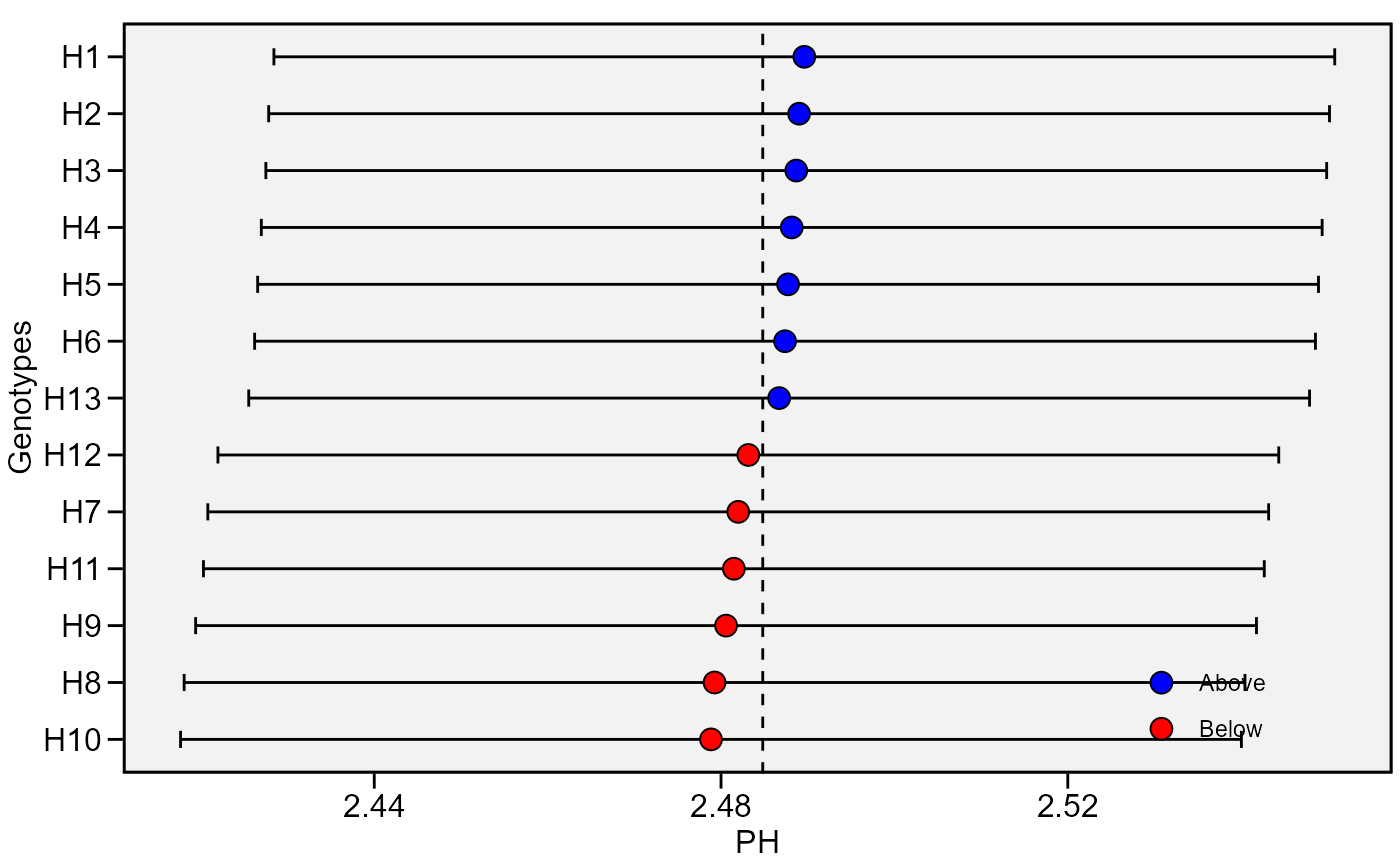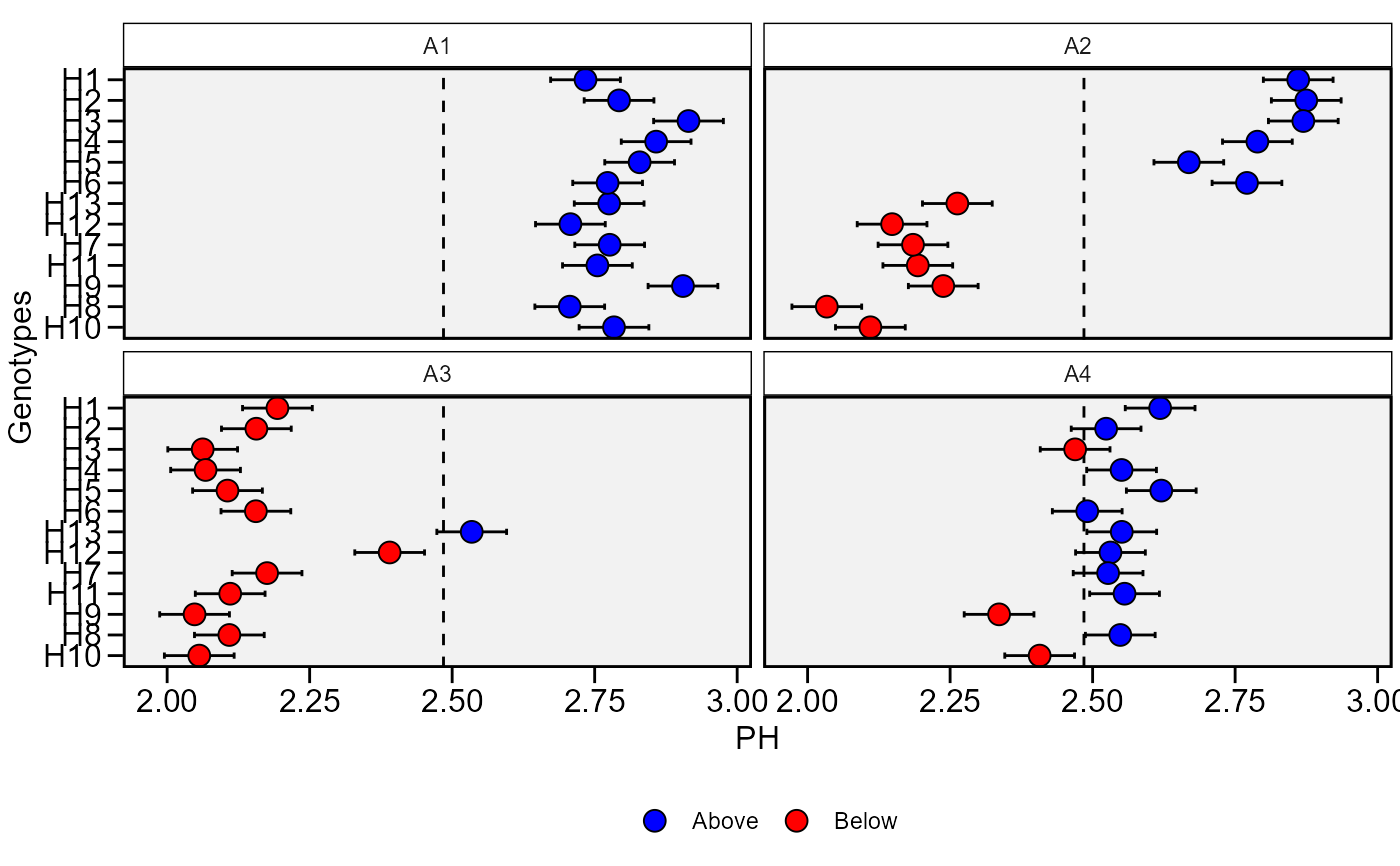Plot the predicted BLUP of the genotypes.

## Usage

plot_blup(
x,
var = 1,
which = "gen",
ncol = NULL,
nrow = NULL,
prob = 0.05,
export = FALSE,
file.type = "pdf",
file.name = NULL,
plot_theme = theme_metan(),
width = 6,
height = 6,
err.bar = TRUE,
size.err.bar = 0.5,
size.shape = 3.5,
size.tex.lab = 12,
height.err.bar = 0.3,
x.lim = NULL,
x.breaks = waiver(),
col.shape = c("blue", "red"),
y.lab = "Genotypes",
x.lab = NULL,
n.dodge = 1,
check.overlap = FALSE,
panel.spacing = 0.15,
resolution = 300,
...
)

## Arguments

x

The waasb object

var

The variable to plot. Defaults to var = 1 the first variable of x.

which

Which plot to shown. If which = "gen" (default) plots the BLUPs for genotypes. To create a plot showing the BLUPs for genotype-environment combinations, use which = "ge".

ncol, nrow

The number of columns and rows, respectively, to be shown in the plot when which = "ge".

prob

The probability error for constructing confidence interval.

export

Export (or not) the plot. Default is TRUE.

file.type

If export = TRUE, define the type of file to be exported. Default is pdf, Graphic can also be exported in *.tiff format by declaring file.type = "tiff".

file.name

The name of the file for exportation, default is NULL, i.e. the files are automatically named.

plot_theme

The graphical theme of the plot. Default is plot_theme = theme_metan(). For more details, see ggplot2::theme().

width

The width "inch" of the plot. Default is 6.

height

The height "inch" of the plot. Default is 6.

err.bar

Logical value to indicate if an error bar is shown. Defaults to TRUE.

size.err.bar

The size of the error bar for the plot. Default is 0.5.

size.shape

The size of the shape (both for genotypes and environments). Default is 3.5.

size.tex.lab

The size of the text in axis text and labels.

height.err.bar

The height for error bar. Default is 0.3.

x.lim

The range of x-axis. Default is NULL (maximum and minimum values of the data set). New arguments can be inserted as x.lim = c(x.min, x.max).

x.breaks

The breaks to be plotted in the x-axis. Default is authomatic breaks. New arguments can be inserted as x.breaks = c(breaks)

col.shape

A vector of length 2 that contains the color of shapes for genotypes above and below of the mean, respectively. Default is c("blue", "red").

y.lab

The label of the y-axis in the plot. Default is "Genotypes".

x.lab

The label of the x-axis in the plot. Default is NULL, i.e., the name of the selected variable.

n.dodge

The number of rows that should be used to render the Y labels. This is useful for displaying labels that would otherwise overlap.

check.overlap

Silently remove overlapping labels, (recursively) prioritizing the first, last, and middle labels.

panel.spacing

Defines the spacing between panels when which = "ge".

resolution

The resolution of the plot. Parameter valid if file.type = "tiff" is used. Default is 300 (300 dpi)

...

Currently not used.

## Value

An object of class gg, ggplot.

plot_scores(), plot_waasby()

## Author

Tiago Olivoto tiagoolivoto@gmail.com

## Examples

# \donttest{
library(metan)
BLUP <- waasb(data_ge2,
resp = PH,
gen = GEN,
env = ENV,
rep = REP)
#> Evaluating trait PH |============================================| 100% 00:00:01

#> Method: REML/BLUP
#> Random effects: GEN, GEN:ENV
#> Fixed effects: ENV, REP(ENV)
#> ---------------------------------------------------------------------------
#> P-values for Likelihood Ratio Test of the analyzed traits
#> ---------------------------------------------------------------------------
#>     model       PH
#>  COMPLETE       NA
#>       GEN 9.39e-01
#>   GEN:ENV 1.09e-13
#> ---------------------------------------------------------------------------
#> All variables with significant (p < 0.05) genotype-vs-environment interaction
plot_blup(BLUP)plot_blup(BLUP, which = "ge")# }